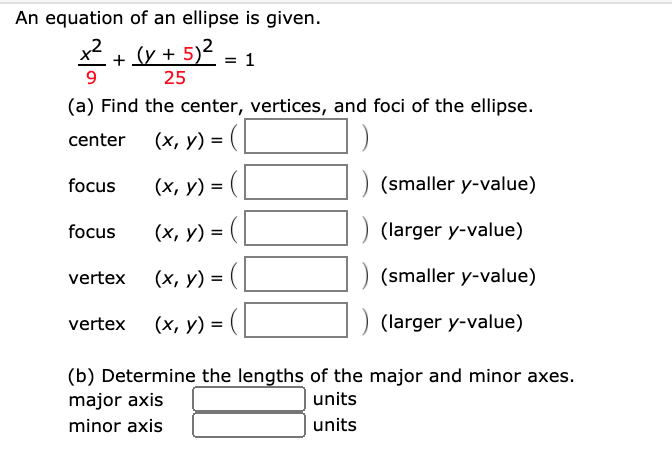# An equation of an ellipse is given. *+v + 5)2. 1 25 (a) Find the center, vertices, and foci of the ellipse. center (x, y) = (L focus (х, у) %3D (smaller y-value) focus (х, у) %3D (larger y-value) vertex (х, у) %3D (smaller y-value) vertex (x, y) = (L ) (larger y-value) (b) Determine the lengths of the major and minor axes. major axis units minor axis | units

Questionhelp_outlineImage TranscriptioncloseAn equation of an ellipse is given. *+v + 5)2. 1 25 (a) Find the center, vertices, and foci of the ellipse. center (x, y) = (L focus (х, у) %3D (smaller y-value) focus (х, у) %3D (larger y-value) vertex (х, у) %3D (smaller y-value) vertex (x, y) = (L ) (larger y-value) (b) Determine the lengths of the major and minor axes. major axis units minor axis | units fullscreen

### Want to see this answer and more?

Experts are waiting 24/7 to provide step-by-step solutions in as fast as 30 minutes!*

*Response times may vary by subject and question complexity. Median response time is 34 minutes for paid subscribers and may be longer for promotional offers.
Tagged in
MathCalculus

### Other